# Unipotent group

Jump to: navigation, search

A subgroupof a linear algebraic groupconsisting of unipotent elements (cf. Unipotent element). Ifis identified with its image under an isomorphic imbedding in a group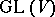of automorphisms of a suitable finite-dimensional vector space, then a unipotent group is a subgroup contained in the set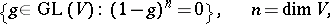of all unipotent automorphisms of. Fixing a basis in, one may identifywith the general linear group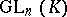, whereis an algebraically closed ground field; the linear groupis then also called a unipotent group. An example of a unipotent group is the group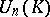of all upper-triangular matrices inwith 1's on the main diagonal. Ifis a subfield ofandis a unipotent subgroup in, thenis conjugate overto some subgroup of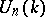. In particular, all elements ofhave ina common non-zero fixed vector, andis a nilpotent group. This theorem shows that the unipotent algebraic groups are precisely the Zariski-closed subgroups offor varying.

In any linear algebraic groupthere is a unique connected normal unipotent subgroup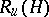(the unipotent radical) with reductive quotient group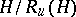(cf. Reductive group). To some extent this reduces the study of the structure of arbitrary groups to a study of the structure of reductive and unipotent groups. In contrast to the reductive case, the classification of unipotent algebraic groups is at present (1992) unknown.

Every subgroup and quotient group of a unipotent algebraic group is again unipotent. If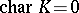, thenis always connected; moreover, the exponential mapping(where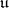is the Lie algebra of) is an isomorphism of algebraic varieties; if, then there exist non-connected unipotent algebraic groups: e.g. the additive group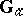of the ground field (which may be identified with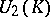) is a-group and so contains a finite unipotent group. In a connected unipotent groupthere is a sequence of normal subgroups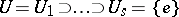such that all quotients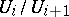are one-dimensional. Every connected one-dimensional unipotent algebraic group is isomorphic to. This reduces the study of connected unipotent algebraic groups to a description of iterated extensions of groups of type.

Much more is known about commutative unipotent algebraic groups (cf. ) than in the general case. If, then they are precisely the algebraic groups isomorphic to; here, the isomorphism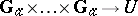is given by the exponential mapping. If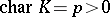, then the connected commutative unipotent algebraic groupsare precisely the connected commutative algebraic-groups. Nowneed not be isomorphic to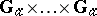: for this it is necessary and sufficient that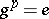for all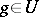. In the general caseis isogenous (cf. Isogeny) to a product of certain special groups (so-called Witt groups, cf. ).

Ifandare connected unipotent algebraic groups and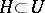, then the variety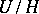is isomorphic to an affine space. Any orbit of a unipotent algebraic group of automorphisms of an affine algebraic varietyis closed in.